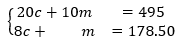# A.2.15.2 Classroom Supplies

A teacher purchased 20 calculators and 10 measuring tapes for her class and paid \$495. Later, she realized that she didn’t order enough supplies. She placed another order of 8 of the same calculators and 1 more of the same measuring tape and paid \$178.50.  This system represents the constraints in this situation:In this situation, what do the solutions to the first equation mean?

What do the solutions to the second equation mean?

For each equation, how many possible solutions are there?

In this situation, what does the solution to the system mean?

Find the solution to the system.

To be reimbursed for the cost of the supplies, the teacher recorded: “Items purchased: 28 calculators and 11 measuring tapes. Amount: \$673.50.”  Write an equation to represent the relationship between the numbers of calculators and measuring tapes, the prices of those supplies, and the total amount spent.

How is this equation related to the first two equations?

In this situation, what do the solutions of this equation mean?

How many possible solutions does this equation have? How many solutions make sense in this situation? Explain your reasoning.